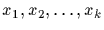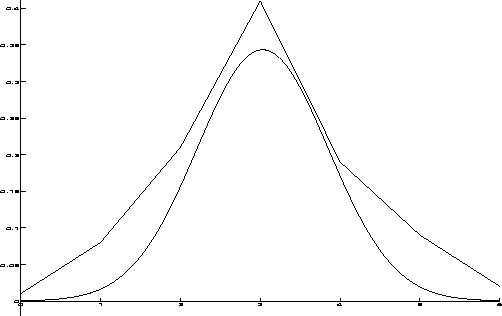Next: More Complicated Plots Up: Creating Plots Previous: Plotting a Function and

Superimposing Plots

It is easy to superimpose different plots by passing multiple functions as a set to DrawPlot.
DrawPlot({}, r)
Each xi must either be a procedure or it must consist of a list of points. The following example compares a set of points given by the list distr with its normal distribution.

>  # the first distribution
> distr := [0.01, 0.08, 0.21, 0.41, 0.19, 0.09, 0.02];

>  # its normal distribution
> mean := 3.03:  variance := 1.35:
> DrawPlot( {distr,
>            x -> exp(-(x-mean)^2/variance) / sqrt(2*Pi*variance)},
>            0..6);
The result is shown in Figure.Gaston Gonnet
1998-09-15